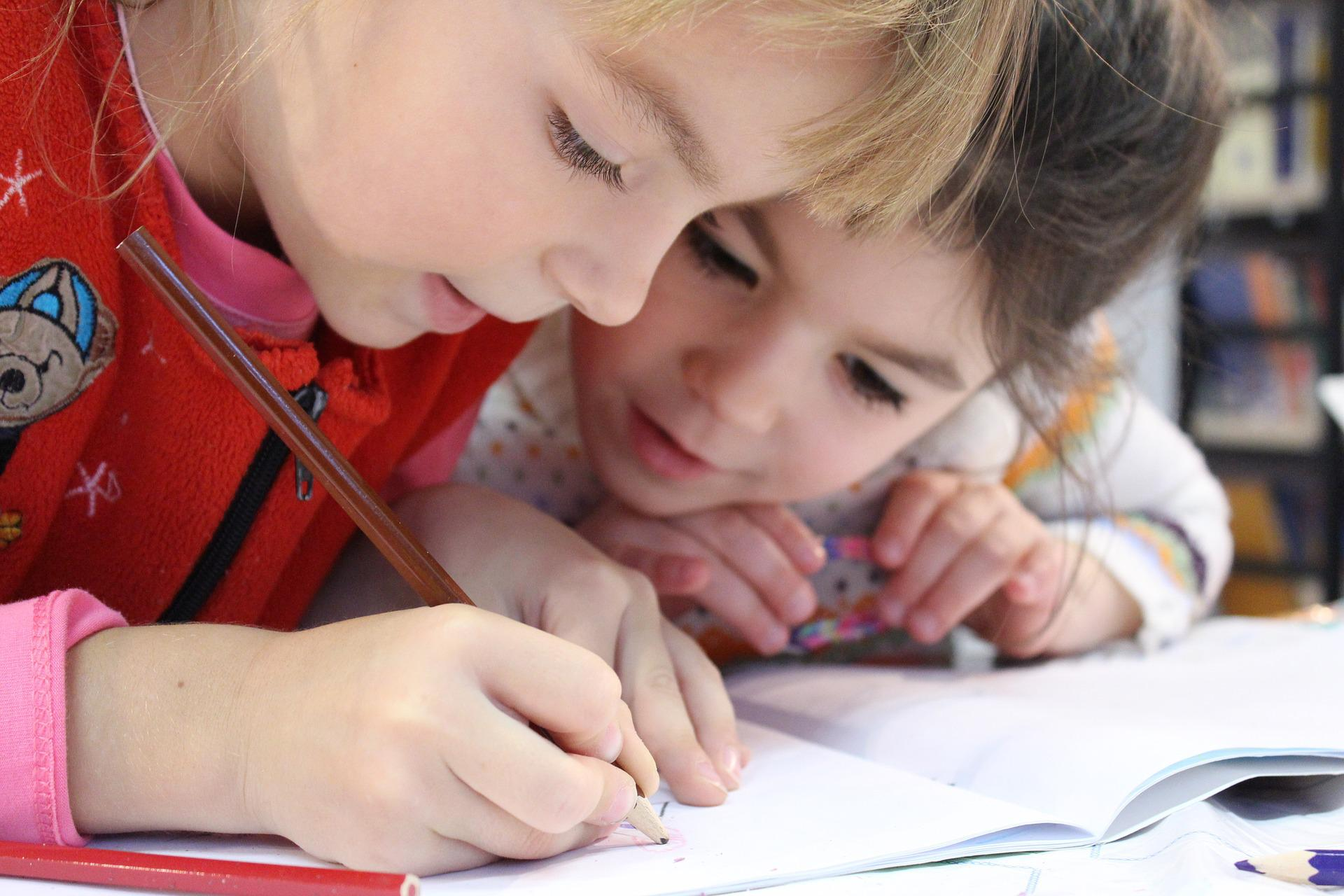Generic selectors
Exact matches only
Search in title
Search in content
Post Type Selectors
post
page# Learn to multiplyOne of the first things children learn in mathematics is multiplication, a method that allows them to add large quantities quickly and easily. Memorizing the multiplication tables can become a real journey. That is why it is so important to introduce them through games and activities. Here are some tips that you can apply to make it easier for the little ones to memorize the multiplication tables:

1. Start with the easiest multiplication tables: the table of 0, 1, 2, 5 and 10. These tables have very basic rules, which children can easily understand:

• Table of 0: any number multiplied by 0 results in 0.
• Table of 1: any number multiplied by 1 results in the same number.
• Table of 2: any number multiplied by 2 results in double that number.
• Table of 5: any number multiplied by 5 ends in 0 or 5.
• Table of 10: any number multiplied by 10 results in the same number accompanied by a 0.

2. Trick boards: there are some tables such as 9 that have a very simple trick to calculate them. In this case, we create two columns of numbers: one in increasing order from 0 to 9 and the other in decreasing order from 9 to 0. If we put both columns together, we get the same result that would be obtained by multiplying the table of 9.

0 9

1 8

2 7

3 6

4 5

5 4

6 3

7 2

8 1

9 0

There is also another way to learn the table of 9, using the hands. With both hands open face down and all fingers extended, the child can find out how much 9 x 2 is by closing the second finger. So the result will always be the number of fingers remaining on both sides of the bent finger. In this case, there would be 1 on the left and 8 on the right, resulting in 18.

3. Multiply by singing: music is a very useful and effective tool for children’s learning. There are numerous resources available to everyone, from YouTube videos to gaming apps.

If you haven’t tried our educational platform yet, you can do it for free by filling out this form
this form.

## More entries

• #### Digital Responsibility

25 de May de 2023
• #### The Benefits of Riddles in Education

25 de May de 2023
• #### Visual aids in the classroom

25 de May de 2023
• #### Music: an ally in the classroom

25 de May de 2023